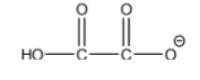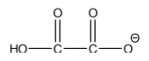# Problem: Answer the following question regarding the hydrogenoxalate ion (structure given below). The Ka2 for the ion is 6.4 x 10–5.When dissolved in water, will this ion produce a basic, acidic, or neutral solution? Circle your answer. Briefly justify your choice using the information given above.a. basicb. acidicc. neutral

###### FREE Expert Solution

We are asked whether this ion produces a basic, acidic, or neutral solution when dissolved in water.

We can observe that the molecule has a full negative charge.###### Problem Details

Answer the following question regarding the hydrogenoxalate ion (structure given below). The Ka2 for the ion is 6.4 x 105.When dissolved in water, will this ion produce a basic, acidic, or neutral solution? Circle your answer. Briefly justify your choice using the information given above.

a. basic

b. acidic

c. neutral